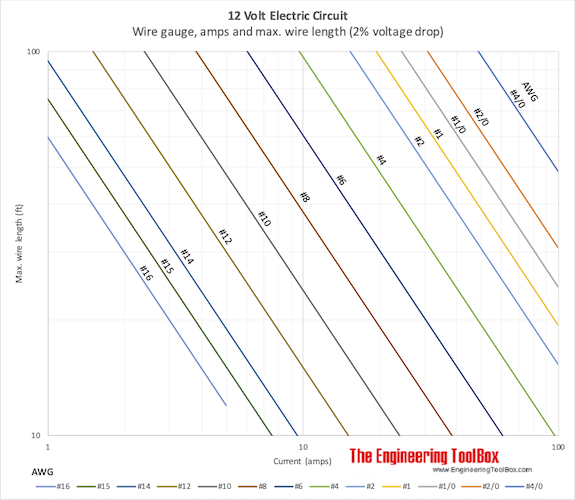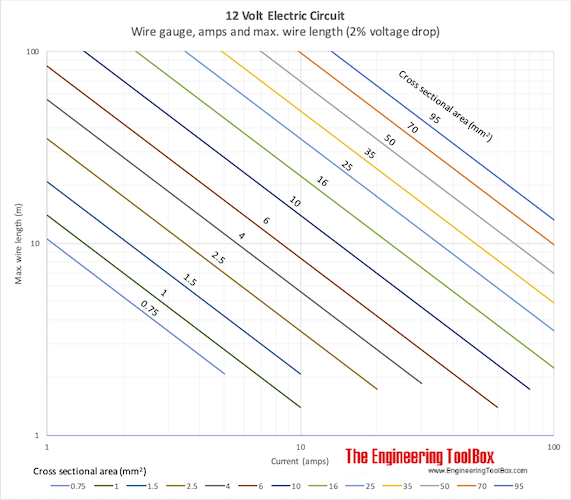Engineering ToolBox - Resources, Tools and Basic Information for Engineering and Design of Technical Applications!

# 12 Volt - Maximum Wire Length vs. Current

## Maximum length of copper wire with 2% voltage drop.

### Maximum Wire Length Calculator

The calculator can be used to calculate maximum length of copper wires. Note that

• for a typical electrical circuit with two wires - one back and one forth - this is the length of the two wires together. The maximum distance between the source and the equipment is half the calculated distance
• in a car where equipment can be grounded to the chassis - the body of the car acts as the negative wire. The electrical resistance in the chassis can normally be neglected and the maximum distance equals the calculated distance

Maximum lengths for copper conductors from power source to loads in 12 volt systems with 2% voltage drop are indicated below:

### Wire Length - feet### Wire Length - meter• double the distance if 4% loss is acceptable
• multiply distance by 2 for 24 volts
• multiply distance by 4 for 48 volts

### Example - Maximum Length of Wire

The current to a light bulb with power 50 W can be calculated with Ohm's law

I = P / U                  (1)

where

I = current (amps)

P = power (watts)

U = voltage (volts)

(1) with values

I = (50 W) / (12 V)

= 4.2 A

From the diagram above the maximum length of the total wire back and forth should not exceed approximately 8 m for gauge #10 (5.26 mm2). By increasing the size of the wire to gauge #2 (33.6 mm2) the maximum length is limited to approximately 32 m.

### Example - Calculate Maximum Wire Length

The electrical resistance in a copper conductor with cross sectional area 6 mm2 is 2.9 10-3 ohm/m. This is close to wire gauge 9.

In a 12V system with maximum 2% voltage drop - and current 10 amps - the maximum total length of the wire back and forth can be calculated with Ohm's law

U = R L I                (2)

where

R = electrical resistance (ohm/m)

L = length of wire (m)

(2) rearranged for L

L = U / (R I)                        (2b)

(2b) with values

L = (12 V) 0.02 / [(2.9 10-3 ohm/m) (10 amps)]

=  8.3 m

## Related Topics

• ### Electrical

Electrical units, amps and electrical wiring, wire gauge and AWG, electrical formulas and motors.

## Related Documents

• ### 12 Volt - Electric Automotive Wiring

Maximum wire lengths vs. currents in the 12 volt electrical system of a car.
• ### 12 Volt - Wire Gauge vs. Amps

Maximum current (amps) in a 12V electrical circuit vs. size (AWG) and length of wire.
• ### AWG - American Wire Gauge Converting Chart

American Wire Gauge (AWG) vs. cross sectional area converter.
• ### Electric Wire - Resistance

Electric resistance in wire made of Copper, Aluminum, Brass, Constantan, Nichrome, Platinum, Silver or Tungsten.
• ### Electrical Formulas

Commonly used electrical formulas like Ohms Law and more.
• ### Piston Engines - Compression Ratios

Cylinder volume and compression ratios in piston engines.

## Engineering ToolBox - SketchUp Extension - Online 3D modeling!

Add standard and customized parametric components - like flange beams, lumbers, piping, stairs and more - to your Sketchup model with the Engineering ToolBox - SketchUp Extension - enabled for use with older versions of the amazing SketchUp Make and the newer "up to date" SketchUp Pro . Add the Engineering ToolBox extension to your SketchUp Make/Pro from the Extension Warehouse !

We don't collect information from our users. More about

## Citation

• The Engineering ToolBox (2010). 12 Volt - Maximum Wire Length vs. Current. [online] Available at: https://www.engineeringtoolbox.com/12-volt-wire-loss-chart-d_1689.html [Accessed Day Month Year].

Modify the access date according your visit.

10.2.10